# Class 9 RD Sharma Solutions – Chapter 17 Constructions- Exercise 17.3

• Last Updated : 26 May, 2021

### Question 1. Construct a Δ ABC in which BC = 3.6 cm, AB + AC = 4.8 cm and ∠B = 60°.

Solution:

Steps of Construction:

1. Draw a line segment BC = 8.6cm.

2. Draw ∠B = 60°.

3. Now, with centre B and radius = 4.8cm, draw an arc that intersects line XB at point D.

4. Join DC.

5. Now, draw a perpendicular of DC that intersects DB at point A.

6. Join AC.

So, Δ ABC is the triangle.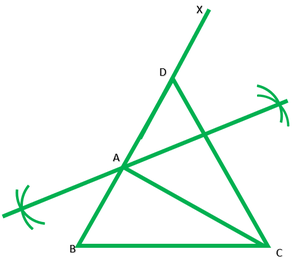### Question 2. Construct a Δ ABC in which AB + AC = 5.6 cm, BC = 4.5 cm and ∠B = 45°.

Solution:

Steps of Construction:

1. Draw a line segment BC = 4.5cm.

2. Draw ∠B = 45°.

3. Now, with centre B and radius = 5.6cm, draw an arc that intersect line BC at point O.

4. Join DC.

5. Now, draw the perpendicular bisector of DC that intersect line BC at point A.

6.Join AC.

So, Δ ABC is the triangle.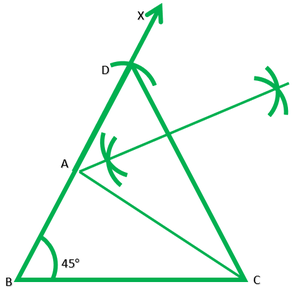### Question 3. Construct a Δ ABC in which BC = 3.4 cm, AB − AC = 1.5 cm and ∠B = 45°.

Solution:

Steps of Construction:

1. Draw  a line segment BC = 3.4cm.

2. Draw ∠B = 45°.

3. Now, with centre B and radius = 1.5cm, draw an arc that intersect line BX at point D.

4. Join DC.

5. Now, draw a perpendicular bisector of DC that intersects line BX at point A.

6. Join AC.

So, Δ ABC is the triangle.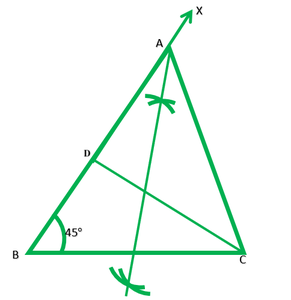### Question 4. Using ruler and compasses only, construct a ΔABC, given base BC = 7 cm, ∠ABC = 60° and AB + AC = 12 cm.

Solution:

Steps of Construction:

1. Draw a line segment BC = 7cm.

2. Draw ∠B = 60°

3. Now, with centre B and radius = 12cm, draw an arc that intersects line BX at point D.

4. Join DC.

5. Now, draw the perpendicular bisector of DC that intersects line BD at point A.

6. Join AC.

So, Δ ABC is the triangle.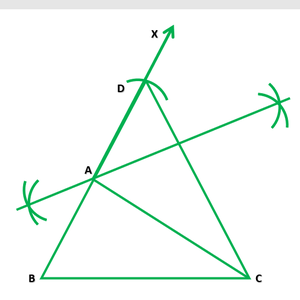### Question 5. Construct a triangle whose perimeter is 6.4 cm, and angles at the base are 60° and 45°.

Solution:

Steps of Construction:

1. Draw a line segment XY = 6.4cm.

2. Draw the angle bisectors of∠EYY = 45°

3. Now, draw the angle bisector of∠BXY and ∠EYX that intersect each other at point A.

4. Draw the perpendicular bisector of lines AX and AY that intersect line XY at points B and C.

5. Join AB and AC.

So, Δ ABC is the triangle.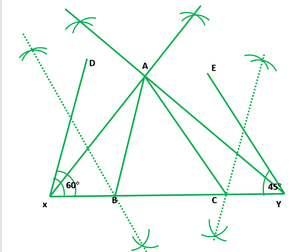### AB + BC + CA = 12 cm, ∠B = 45° and ∠C = 60°.

Solution:

Steps of Construction:

1. Draw a line segment XY = 12cm.

2. Draw∠X = ∠B = 45° and ∠Y = ∠C = 60°

3. Now, draw the angle bisectors of angles of DXY and EYX that intersects each other at point A.

4. Draw the perpendicular bisector of AX and AY that intersect line XY at points B and C.

5. Join AB and AC.

So, Δ ABC is the triangle.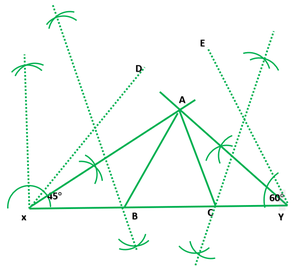### Question 7. Construct a right-angled triangle whose perimeter is equal to 10 cm and one acute angle equal to 60°.

Solution:

Steps of Construction:

1. Draw a line segment XY = 10cm.

2. Draw ∠X = ∠B = 90 and ∠Y = ∠C = 60

3. Now, draw the angle bisector of ∠DXY and ∠EXY that intersect each other at point A.

4. Draw the perpendicular bisectors of AX and AY which intersects line XY at points B and C.

5. Join AB and AC.

So, Δ ABC is the triangle.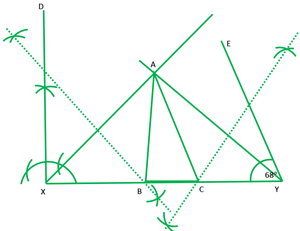### Question 8. Construct a triangle ABC such that BC = 6 cm, AB = 6 cm and median AD = 4 cm.

Solution:

Steps of Construction:

1. Draw a line segment BC = 6 cm

2. Now, take mid-point D of line BC.

3. With centre B and D and radius 6cm and 4cm, draw two arcs that intersects each other at point A.

4. Now, join AB, AD, and AC.

So, Δ ABC is the triangle.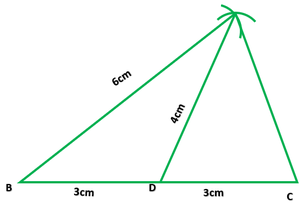### Question 9. Construct a right triangle ABC whose base BC is 6 cm and the sum of hypotenuse AC and other side AB is 10 cm.

Solution:

Steps of Construction:

1. Draw a line segment BC = 6cm.

2. Now, at point B draw and angel of 90°

3. Now, with centre B and radius = 10cm, draw an arc that intersect line XB at point D.

4. Join DC.

5. Draw the perpendicular bisector of DC that intersects line DB at point A.

6. Join AC.

So, Δ ABC is the triangle.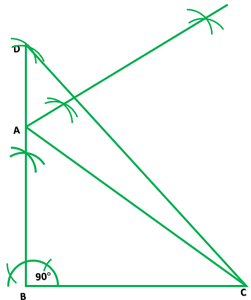### Question 10. Construct a triangle XYZ in which ∠Y = 30°, ∠Z = 90° and XY + YZ + ZX = 11.

Solution:

Steps of Construction:

1. Draw a line segment AB = 11cm.

2. Draw ∠A =∠Y = 30 and ∠B = ∠Z = 90

3. Now, draw the angle bisectors of ∠DAB and ∠EBA that intersect each other at point X.

4. Draw the perpendicular bisectors of XA and XB that intersect line AB at points Y and Z.

5. Join XY and XZ.

So, Δ XYZ is the triangle.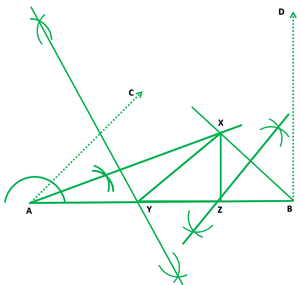My Personal Notes arrow_drop_up